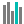#NORM.DIST (PQL - xl)

This function returns the normal cumulative distribution for the specified mean and standard deviation

• Library: PQL \ Spreadsheet \ Statistical
• Compatibility: Any content (regardless of data source) in the Tabulate spreadsheet module
• Solve: This function can be used with the Solve plug-in: both as part of the objective function and constraint functions

#### Syntax

NORM.DIST(x, mean, standard dev, cumulative)

##### Function Arguments
 Name Description Type Optional x Value for which to find the distribution Number mean Arithmetic mean of the distribution Number standard dev Standard deviation of the distribution Must be greater than zero Number cumulative Set to TRUE to return the cumulative distribution function. Set to FALSE to return the probability mass function StringOrNumber